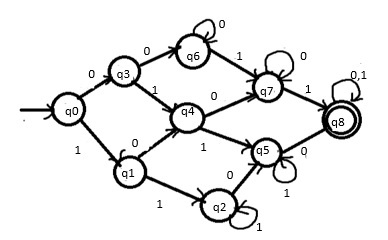# Design a DFA of a string with at least two 0’s and at least two 1’s

A Deterministic Finite automaton (DFA) is a 5-tuples

M=(Q, Σ, δ,q0,F)

Where,

• Q : Finite set called states.
• Σ : Finite set called alphabets.
• δ : Q × Σ → Q is the transition function.
• q0 ϵ Q is the start or initial state.
• F : Final or accept state.

## Problem

Construct DFA of a string with at least two 0’s and at least two 1’s.

## Solution

The language generated based on the given condition over the alphabet Σ ={0,1) is −

L={0011,001011,0001010,0011001,010101,……}

The given language accepts at least two zero’s means it can accept two or more than two zero’s and at least two one’s means it accepts two or more than two one’s.

Suppose,

• Input − 00010
• Output − string rejected

Because, the given input does not have at least two’s.

Even though it has at least zeros, it won’t accept the string.

To accept the string both conditions are satisfied, if one fails the string will not be accepted by the machine.

• Input − 001001001
• Output − string accepted

Now construct the DFA for given inputs −

StateNumber of zero’sNumber of one's
→ q000
q101
q20> =2
q310
q411
q51> =2
q6> =20
q7> =21
*q8> =2> =2

The DFA will be as follows −## Explanation

If input is 1 then the number of 1 increases to 1. Move to state q1. If input is 0 then the number of 0 increases to 1. Move to state q3.

If input is 1 then the number of 1 increases to 2. Move to state q2. If input is 0 then the number of 0 increases to 1. Move to state q4.

If input is 1 then the number of 1 always increases by 1. And then remain in the same state. If input is 0 then the number of 0 increases to 1. Move to state q5.

If input is 1 then the number of 1 increases to 1. Move to state q4. If input is 0 then the number of 0 increases to 2. Move to state q6.

If input is 1 then the number of 1 increases to 2. Move to state q5. If input is 0 then number of 0 increases to 2. Move to state q7.

If input is 1 then the number of 1 always increases by 1. And then remain in the same state. If input is 0 then the number of 0 increases to 2. Move to state q8

If input is 1 then the number of 1 increase to 1. Move to state q7. If input is 0 then the number of 0 keeps increasing by 1. And then remain in the same state.

If input is 1 then the number of 1 increase to 2. Move to state q8. If input is 0 then the number of 0 keeps increasing by 1. And then remain in the same state.

If input is 1 then the number of 1 always increases by 1. And then remain in the same state. If input is 0 then the number of 0 keeps increasing by 1. And then remain in the same state.

Finally, if the string is finished, then it is ACCEPTED.